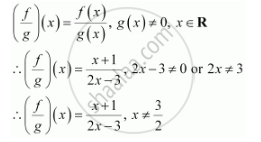CBSE (Arts) Class 11CBSE
Share

# Let F, G: R → R Be Defined, Respectively By F(X) = X + 1, G(X) = 2x – 3. Find F + G, F – G And F/G - CBSE (Arts) Class 11 - Mathematics

ConceptAlgebra of Real Functions

#### Question

Let fgR → R be defined, respectively by f(x) = + 1, g(x) = 2x – 3. Find f + gf – g and f/g

#### Solution

fgR → is defined as f(x) = + 1, g(x) = 2x – 3

(f + g) (x) = f(x) + g(x) = (x + 1) + (2x – 3) = 3x – 2

∴(f + g) (x) = 3x – 2

(f – g) (x) = f(x) – g(x) = (x + 1) – (2x – 3) = x + 1 – 2x + 3 = – x + 4

∴ (f – g) (x) = –x + 4Is there an error in this question or solution?

#### APPEARS IN

NCERT Solution for Mathematics Textbook for Class 11 (2018 to Current)
Chapter 2: Relations and Functions
Ex. 2.40 | Q: 7 | Page no. 46

#### Video TutorialsVIEW ALL 

Solution Let F, G: R → R Be Defined, Respectively By F(X) = X + 1, G(X) = 2x – 3. Find F + G, F – G And F/G Concept: Algebra of Real Functions.
S